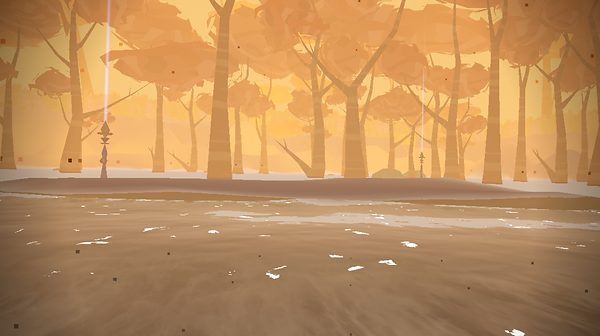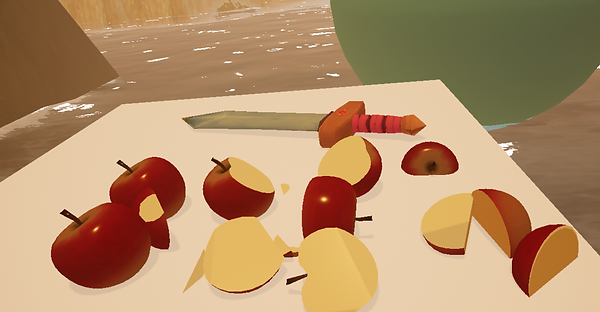top of page# Visual Effects & VR Sickness# VR Locomotion

## Virtual Worlds aims to create an immersive experience for players. Therefore the game's locomotion system makes no use of teleportation, as this was discovered to create a disconnect between a player and the virtual environment. Instead the game makes use of analogue and arm swing locomotion. These methods of movement combined with the designed visual effects (Dot Effect/ Dynamic FOV), have created a locomotion system that gives the player freedom of movement with reduced sickness.# Mechanics

## ​

The equation for working out the turning velocity was:

• V1 = box collision centre.

• V2 = hands centre.

• V2-V1 = distance between two points (V3).

• √ (V3x ² + V3y ² +V3z ²) = magnitude (V4).

• (V4) = Turning Velocity Scalar.

The turning velocity scalar was then used in this equation to add turning to the gliding:

• V1 = box collision centre.

• V2 = hands centre.

• V3 = Turning Velocity Scalar.

• V4 = Glide Direction (Forward Vector).

• ---

• V2-V1 = distance between two points (V4).

• √ (V4x ² + V4y ² +V4z ²) = magnitude (V5).

• (V4)/(V5) = Normalised distance (V6).

• V6 * (V4*V3) = Position Offset (V7).

## ​## Procedural Mesh - Apples# Asset Management

## Below you can find some examples of models and textures designed for this project.bottom of page